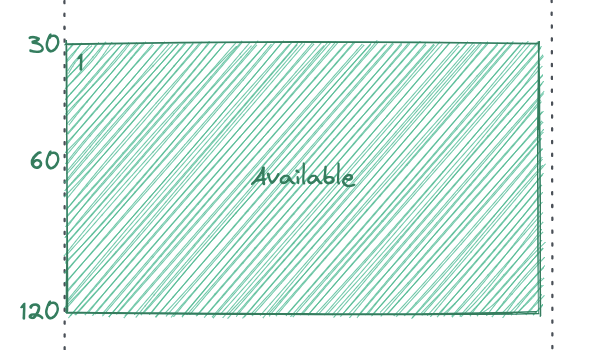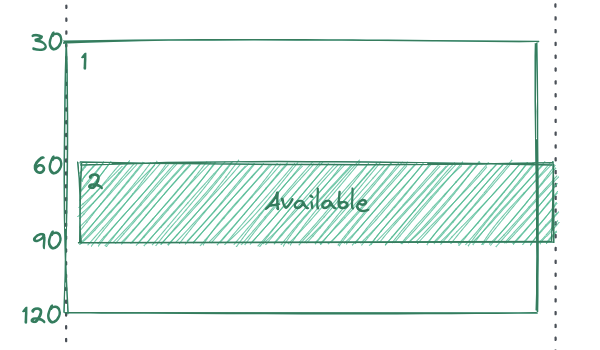Live Demo

Repository

### Too many events all at once¶

Let’s assume that we want to stack two calendar blocks, `block_1` starts at 12:30 AM and ends at 02:00 AM, and `block_2` starts at 01:00 AM and ends at 01:30 AM. To simplify things however, let’s just use their start and end times as minutes i.e. an event that starts at 12:30 AM would just be starting at minute 30.

To display the blocks we’re going to use their start time as a top offset. Assuming that the day starts at minute 0, a block that starts at minute 30 will have a 30 px offset from the top. Keeping the page height in sync with the minutes in the day will make the offset math convenient.### The recursion trap¶

Self-referential data types are more intuitive for modeling relationships between calendar nodes.

All calendar events can be represented by a handful of properties:

``````// using typescript to write pseudocode
interface CalendarBlock {
id: number
startMinute: number
endMinute: number
blockType: 'busy' | 'available'
children: CalendarBlock[]
}
``````

Forward traversal can be achieved elegantly by:

``````currentBlock = currentBlock.children;
``````

However, the Rust compiler needs to know the size of objects at compile time. As it’s impossible to determine the size of self-referential data types, we have to use `RefCell`s to model the `CalendarBlock`s.

Rust implementation of self-referential CalendarBlock:

``````enum CalendarBlockType {
Busy,
Available,
}

struct CalendarBlock {
id: u32,
startMinute: u32,
endMinute: u32,
blockType: BlockType
children: Vec<RefCell<CalendarBlock>>
}
``````

I didn’t want to do that as it seemed “un-idiomatic”, and decided to use a separate adjacency list using Petgraph to store the calendar block tree instead.

Petgraph has a clean API and we’re going to use a directed graph to represent the calendar block tree.

So now the we can get rid of the `RefCell`.

``````struct CalendarBlock {
startMinute: u32,
endMinute: u32,
blockType: BlockType,
}

type Weight = usize;
pub struct CalendarBlockTree {
root_idx: NodeIndex,
}

fn main() {
let calendar_block_1 = CalendarBlock {
id: 1,
startMinute: 30,
endMinute: 120,
blockType: CalendarBlockType::Available,
};
let calendar_block_2 = CalendarBlock {
id: 2,
startMinute: 60,
endMinute: 90,
blockType: CalendarBlockType::Available,
};

// add_edge takes the source, destination, and weight as params

// now that the nodes and edges are in place we can instantiate our CalendarBlockTree
let calendar_block_tree = CalendarBlockTree {
root_idx: node_1_idx,
};
}
``````

### Traversal¶

Petgraph’s `edges_directed` API returns an iterator over `EdgeReference`. `EdgeReference` has methods to retrieve the `source`, `target`, and `weight` of a particular edge. We can use `edges_directed` to find edges in both directions forward and backward (used later).

``````let forward_neighbors_list: Vec<petgraph::graph::EdgeReference<usize>> = self
.edges_directed(destination, petgraph::Direction::Outgoing)
.collect();

// unwrap is a story we're not going to tell today
let node_index = forward_neighbors.first().unwrap();

``````

Forward traversal is useful for calculating stack position, and backward traversal is useful for calculating the sub-tree depth (also called height) of each node.

Our first algorithm does not require the sub-tree depth so we’ll start there.

### Stacking blocks¶

When populating the tree I had to make sure that the calendar blocks are added in the right place. For simplicity I populate the tree every time a block is added/moved. As the blocks get sorted before the tree is populated we can create the correct hierarchy using the following rules:

• compare the new block with existing blocks starting from the root
• if the events overlap the event that starts later will be deeper in the tree (as we start with a sorted list this will always be the new block)
• if the events do not overlap we check the next event in the list of neighbors for an overlap
• if no overlaps found the event gets added at the end (sorting helps here as well)

To determine whether a calendar block overlaps with another I added a method to the `CalendarBlock` struct:

``````impl CalendarBlock {
pub fn does_overlap(&self, block: CalendarBlock) -> Option<CalendarBlockOverlap> {
if self.start_minute >= block.end_minute || self.end_minute < block.start_minute {
return None;
}

if self.start_minute > block.start_minute
|| (self.start_minute == block.start_minute && self.end_minute <= block.end_minute)
{
return Some(CalendarBlockOverlap::GetsSwallowed);
}

Some(CalendarBlockOverlap::Swallows)
}
}
``````

### Calendar tree with stack position¶

This section is a WIP.